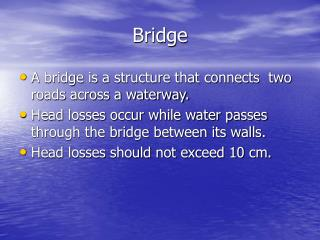DownloadDownload PresentationBridge

# Bridge

Download Presentation## Bridge

- - - - - - - - - - - - - - - - - - - - - - - - - - - E N D - - - - - - - - - - - - - - - - - - - - - - - - - - -
##### Presentation Transcript

1. Bridge • A bridge is a structure that connects two roads across a waterway. • Head losses occur while water passes through the bridge between its walls. • Head losses should not exceed 10 cm.

2. ≤ 10 cm Water depth d Longitudinal section in a bridge.

3. B 0.75 m S d b Cross section in a bridge

4. Hydraulic Design • Hydraulic design is conducted to obtain the number of vents (n) and the span of the vent (s) • The selection is made such that the head losses does not exceed 10 cm. • A simple design can be achieved following the next steps:

5. Assume n, then calculate S as follows: • Adjust S to a practical value (S ≤ 5.0 m) • Calculate the velocity through the bridge Where Q is the discharge in m3/sec, V is the velocity in m/sec and Aw is the water area.

6. Aw = n . S . d Where d is the water depth. • Head losses is calculated as follows Where Hl is head loss (m), g is gravitational acceleration (9.81 m/sec2), C is a constant.

7. C = 0.72 for S ≤ 2.0 m • C = 0.82 for S ≤ 4.0 m • C = 0.92 for S > 4.0 m

8. Example: Design a bridge across a waterway having a discharge of 18 m3/sec. The bottom width is 15 m and the top width is 21 m and the water depth is 3 m. • Sol. Assume n = 5 S = (21-3)/5 = 3.6 m Aw = (15+21)/2 * 3 = 54 m2

9. v = 18/54 = 0.33 m/sec Av= 5 * 3.6 * 3 = 54 m2 Check of Heading Up Since Aw = Av .. Hu = 0 Hu < 0.1 m … OK